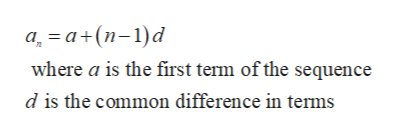# An object in free fall is dropped from a tall cliff. It falls 16 ft in the first second, 48 ft in the second second, 80 ft in the third second, and so on.a. Write a formula for the nth term of an arithmetic sequence that represents the distance dn (in ft) that the object will fall in the nth second.b. How far will the object fall in the 8th second?c.What is the total distance that the object will fall in 8 sec?

Question
7 views

An object in free fall is dropped from a tall cliff. It falls 16 ft in the first second, 48 ft in the second second, 80 ft in the third second, and so on.

a. Write a formula for the nth term of an arithmetic sequence that represents the distance dn (in ft) that the object will fall in the nth second.

b. How far will the object fall in the 8th second?

c.What is the total distance that the object will fall in 8 sec?

check_circle

Step 1

Step 1:

The object fall in the first second = 16ft

The object fall in the second second = 48ft

The object fall in the third second = 80ft

So, it become a arithmetic sequence with common difference 32.

The formula for the nth term of an arithmetic sequence is:help_outlineImage Transcriptioncloseа, —а+(п-1)d where a is the first term of the sequence d is the common difference in terms fullscreen
Step 2
1. b) the above formula represent the distance that falls on every second after n seconds:
Step 3

The total distance that the obje...

### Want to see the full answer?

See Solution

#### Want to see this answer and more?

Solutions are written by subject experts who are available 24/7. Questions are typically answered within 1 hour.*

See Solution
*Response times may vary by subject and question.
Tagged in

### Algebra